### Home > MC2 > Chapter 1 > Lesson 1.1.4 > Problem1-28

1-28.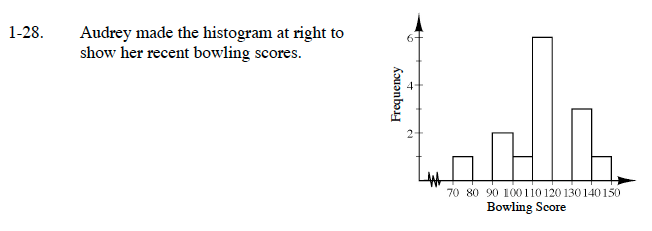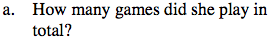Each bar height represents the frequency and tells you how many games had a score that fell between the lower and upper value of that bar. For example, 1 game had a score that fell between 70 and 79. Try to add up all the frequencies to find the total number of games.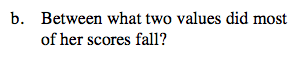Which bar is the tallest?
Which scores do these bars represent?

Between what score range do at least half of her games fall?
Examine the graph around the tallest bars to see where the range of bars is where most of the score values fall.

Most of Audrey's scores were between 90 and 119.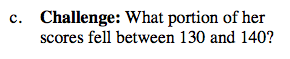Examine the bar highlighted in the graph representing the games played with scores between 130 and 139.
How many games were played in this interval?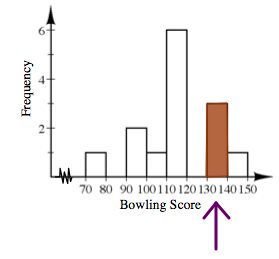3 out of the total 14 scores fall between 130 and 139.

$\text{This can be written as: } \frac {3}{14}$